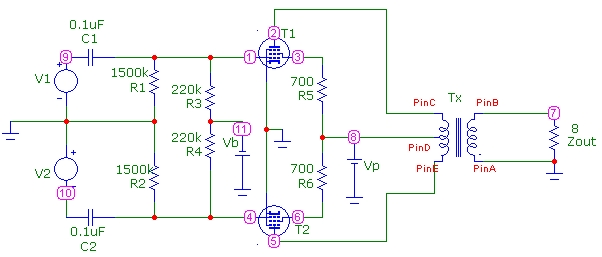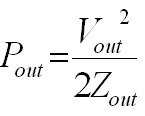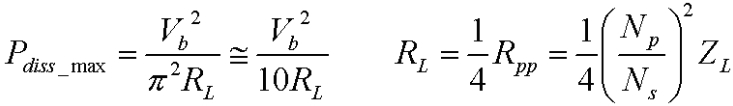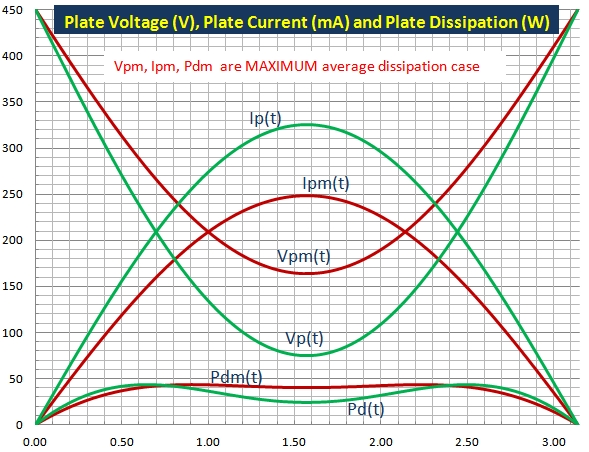# Push-Pull Tube Amplifier Simulation

Mar 02, 2016The results below present circuit simulations for a push-pull pentode 2-tube power amplifier. The Microcap simulator was used to produce the graphs for a signal frequency of 1kHz. The power tubes are standard 6L6 pentodes. These circuit values correspond approximately to the 65 Fender Twin Reverb Amp but with two tubes (instead of 4) and with an 8 Ω speaker load (instead of the 4Ω load for 4 tubes). The plate supply voltage Vp is 450VDC, the control-grid bias voltage is -44VDC, and the quiescent plate current per tube is 36mA. The zero-signal plate dissipation per tube is 16.2W. The peak control-grid signal voltage is 37Vpeak. The center-tapped output transformer is characterized by L1 = L2 = 14.4H (half side of primary) and L3 = 0.1H (output secondary) corresponding to a full turns ratio of N1/N2=24. Except for finite transformer inductances, the simulator model assumes an ideal transformer with zero DC resistance and no internal losses. The schematic diagrams below show component values and zero-signal DC voltage levels. The plate characteristic shows the single-tube load line for the 6L6 tube for the maximum power condition with maximum plate voltage swing corresponding to the grid voltage Ec1 swinging to zero. The screen-grid voltage for this circuit is close to 450.## Maximum Power Output

The power delivered to the transformer primary can be calculated from the voltage-swing (or current-swing) along the load-line for the half-cycle that each tube in the push-pull circuit contributes. The effective load impedance RL that each tube experiences over it's conducting half-cycle, which is the slope of the load-line, is just 1/4 of the full plate-to-plate impedance Rpp of the transformer primary RL = 1/4*Rpp because each half of the center-tapped transformer has half the turns. Since Rpp = (N1/N2)^2*Zout, and with N1/N2=24 and Zout=8Ω for this study, Rpp=4.6kΩ and RL = 1.15kΩ. Since the peak voltage swing for a half cycle is (Vp-Vmin) and the corresponding peak current swing is Imin, the averaged power delivered to the transformer primary is:which, for an ideal transformer, must equal the output power delivered to the (speaker) load, where Vout is the peak output voltage:From the load line and also the simulation curves for plate voltage and plate current, (Vp-Vmin) = 375V and Imax = 320mA. Therefore the power delivered to the transformer primary (combination of both tubes) is Pp = 60W. The simulation below shows Vout=31Vpeak. Therefore the output power into the 8Ω load is Pout= 60W in agreement with the power delivered to the transformer primary for the ideal transformer model used in the simulation.The plate voltage curve shows some distortion in the slope corresponding to cross-over distortion centered around Vp=450V.## Plate Power Dissipation

The plate power dissipation at zero signal is the product of the quiescent plate voltage 450V and the plate current 36mA or 16.2W as shown in the zero signal curves below. Under the maximum signal swing, the plate power dissipation of the tube is the average plate power over a complete cycle. For about half this cycle, the tube is not conducting and therefore dissipates no power. The instantaneous plate power dissipation curve below, for a single tube, shows that the instantaneous power goes through a peak and decreases as expected from theory. Averaging the power over a full cycle yields the dynamic plate dissipation for each tube of ~ 18W, within the maximum plate dissipation specification for the 6L6 tube.Alternatively, the plate dissipation can be calculated from the total power supplied by Vp minus the output power delivered to the speaker load minus the power delivered to the screen grid circuit (less than 1 W). The instantaneous power plots over 2 cycles are shown below. The upper result is the instantaneous power dissipated by both tube plates. The average value over a cycle divided by 2 gives ~ 18W for the average dynamic plate dissipation of each tube, in agreement with the result above:It is interesting to compare the simulation results above with basic pentode plate dissipation theory (RCA Receiving Tube Manual) which predicts an average plate dissipation per tube of:With Imax = 320mA and (Vb-Vmin) = 375V, Pdiss=15.8W per tube, somewhat lower than the 18W result from the simulation. The MAXIMUM possible average plate dissipation results for a plate voltage swing of (Vb-Vmin)=2/Sqrt(π)Vp ~ 0.64Vp = 288V, considerably less than the design 375V swing used for maximum power output in the simulation above. The corresponding maximum possible average plate dissipation is:or 17.6W per tube for this circuit. Therefore, this maximum plate-dissipation estimate using basic theory provides a very close estimate to the actual plate dissipation predicted by a numerical simulator. The green curves below show the instantaneous plate voltage Vp(t), current Ip(t) and plate dissipation Vp(t)*Ip(t) over a half-cycle for maximum output power conditions with a 375V plate-voltage swing. The Pd(t) plate dissipation for a single tube agrees closely with the simulation curve above. Note that the basic-theory curve assumes an exact half-cycle of conduction. (The more exact simulation curve above shows the dissipated power extends into the non-conducting half-cycle due to the finite operating-point bias current). The red curves are for the maximum average plate-dissipation case with a smaller plate-voltage swing of 288V. It is obvious from the instantaneous plate-dissipation curve Pdm(t) for the smaller plate voltage swing that the plate dissipation averaged over a half-cycle is higher than for the larger voltage swing case Pd(t). Since the tube conducts for only this half-cycle, the average plate dissipation power is half the time-average of the instantaneous Pd(t) curve displayed below. This is equivalent to the Pdiss(each tube) equation above:References